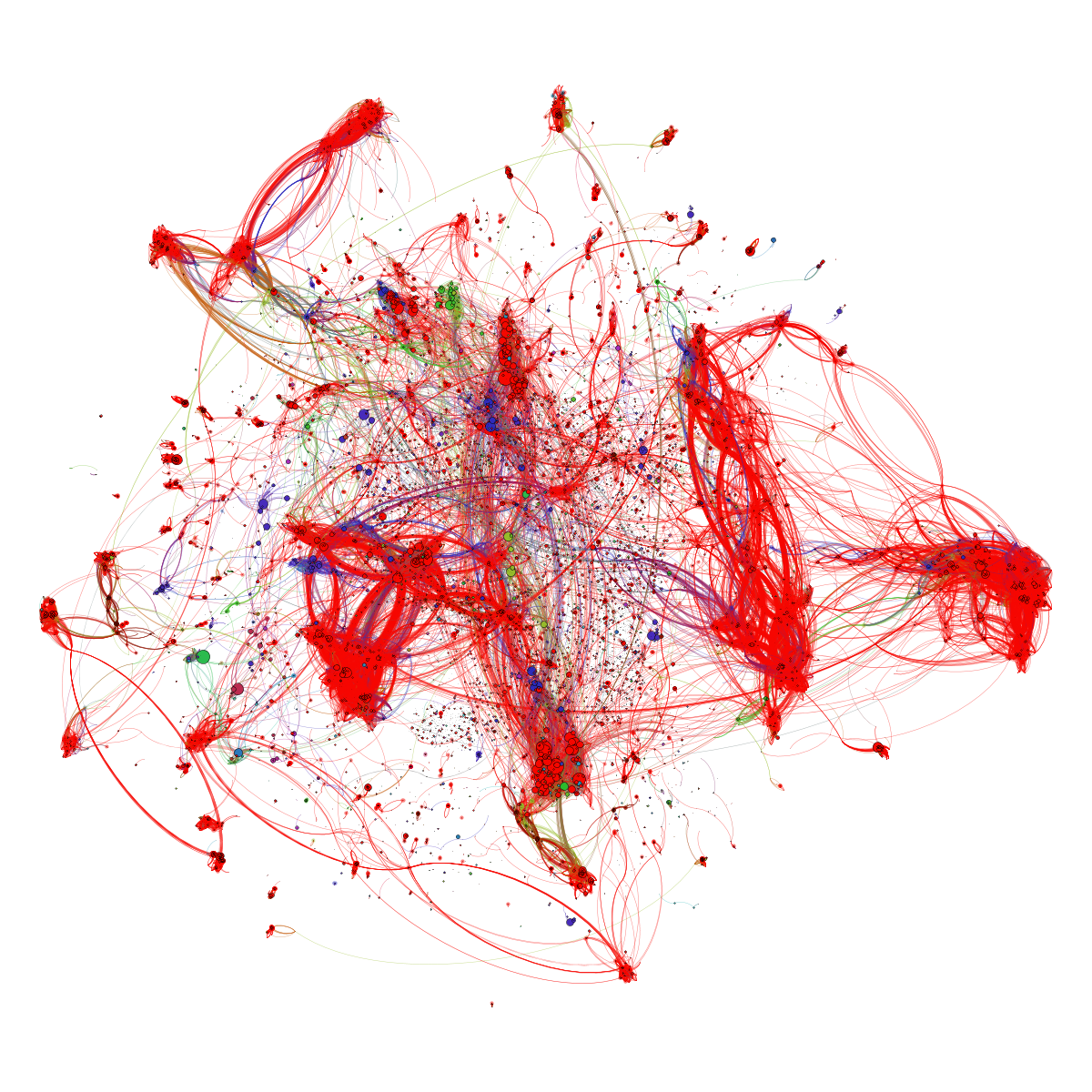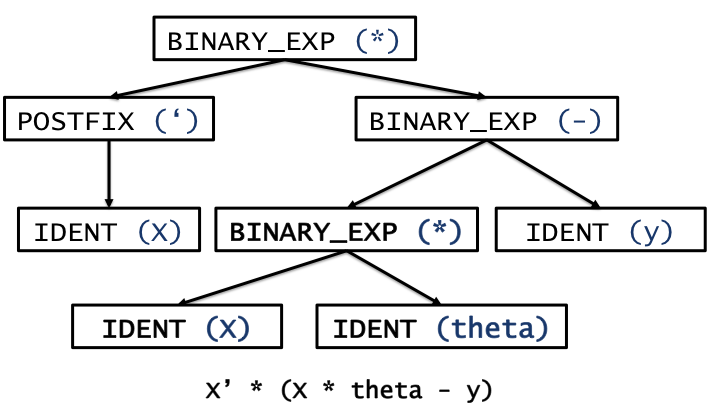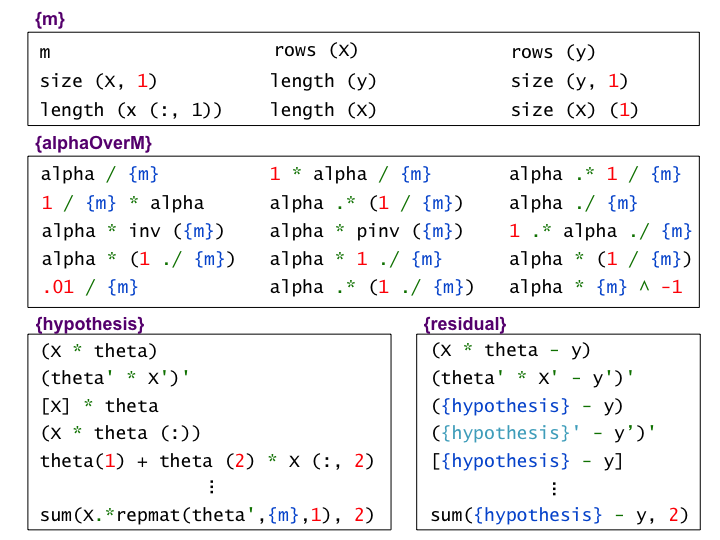### Code Webs - Visualizing 40,000 student code submissionsProject team: Jonathan Huang, Chris Piech, Andy Nguyen, and Leonidas Guibas,
with special thanks to Andrew Ng and Daphne Koller for making the data available to us.

Abstract Art?

Not quite! The above figure is the landscape of ~40,000 student submissions to the same programming assignment on Coursera's Machine Learning course. Nodes represent submissions and edges are drawn between syntactically similar submissions. Colors correspond to performance on a battery of unit tests (with red submissions passing all unit tests).
In particular, clusters of similarly colored nodes correspond to multiple similar implementations that behaved in the same way (under unit tests).

** (For those curious, this particular programming assignment asked students to implement gradient descent for linear regression in Octave).

Here's how we made it:

1. We parsed each of 40,000 student submissions into an Abstract Syntax Tree (AST) data structure by adapting the parsing module from the Octave source code. ASTs allow us to capture the structure of each student's code while ignoring irrelevant information such as whitespace and comments (as well as, to some extent, variable names). Part of the AST might correspond to a mathematical expression, for example, and may look as follows:2. We next computed the tree edit distance between every pair of unique trees, which counts the minimum number of edit operations (e.g., deletes, inserts, replaces) required to transform one tree into the other
3. Reasoning that small edit distances between ASTs are meaningful while larger distances less so, we finally dropped edges whose edit distances were above a threshold and and used gephi to visualize the resulting graph.

See the paper that we presented at MOOCshop 2013 for more details on analyzing a large collection of code submissions using edit distance:

Also see Ben Lorica's blog post, and Hal Hodson's article at New Scientist about this work!

Bonus: we can use big data to discover equivalent ways of coding the same thing!

We have recently developed an automatic data-driven method for discovering code snippets that are semantically equivalent in the context of a homework problem. Below are a few examples of equivalence classes that we can learn from data for the same gradient descent for linear regression problem. Note, for example, that we discover that when a student wrote X*theta for the linear regression problem, she could have equivalently written (theta'*X')'If you want to find out how we did this, read our research paper that describes the details of automatic semantic equivalence discovery and its applications to providing scalable feedback in a MOOC:

But what is it good for?

Well we have a lot of ideas! One thing that we did, for example, was to apply clustering to discover the ``typical'' approaches to this problem. This allowed us to discover common failure modes in the class, but also gave us a way to find multiple correct approaches to the same problem. Stay tuned for more results from the codewebs team!